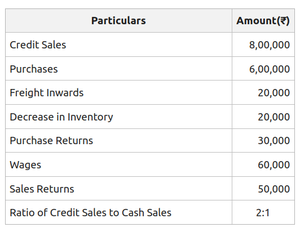Open in App
Not now

# Gross Profit Ratio: Meaning, Formula, Significance and Examples

• Last Updated : 10 Oct, 2022

Financial Statements are prepared to know the profitability and financial position of the business in the market. These financial statements are then analyzed with the help of different tools and methods. Ratio Analysis is one of the methods to analyse financial statements. The relationship between various financial factors of a business is defined through ratio analysis.

Gross Profit ratio is a financial metric, which establishes a relationship between the gross profit of a company and its net revenue from operations. It is used to determine the profit earned by a firm after bearing all its direct expenses, i.e., the expenses directly tied to production. This ratio is used to determine the earning efficiency of the firm.

Gross Profit Ratio is one of the profitability ratios in accounting theory and practice. Profitability ratios are the financial metric employed in order to measure a firm’s ability to generate earnings relative to the turnover, capital employed, assets held, operating costs, etc.

Generally, a higher gross profit ratio indicated an increase in the profit margin. Gross profit ratio can be compared with the previous year’s ratio of the firm or with similar firms to see if it is up to the mark.

• Gross Profit: It refers to the profit earned after deducting all the expenses directly related to production from the net revenue earned from operations. Such profit normally accounts for variable costs and not fixed ones.
• Net Sales: It refers to the revenue earned by the firm by selling its products after adjusting all kinds of sales returns, discounts, allowances to the customers, etc.

### Formula:where,

Gross Profit = Net Revenue from Operations – Cost of Goods Sold

Net Revenue from Operations = Gross Revenue – Sales Return – Discount – Allowances

or

Net Revenue from Operations = Cash Revenue from Operations + Credit Revenue from Operations – Return Revenue from Operations

Cost of Revenue from Operations (C.O.G.S.) = Cost of Material Consumed + Purchase of Stock-in-trade + Changes in Inventories of Finished Goods and Work-in-progress + Direct Expenses

### Significance:

Gross Profit Ratio is used to ascertain the amount of profit available in hand to cover the firm’s operating expenses. A higher gross profit ratio indicated an increase in the profit margin. Gross profit ratio can be compared with the previous year’s ratio of the firm or with similar firms to ascertain the growth. This ratio is also an important measure to know how efficiently an establishment uses labour and supplies for manufacturing goods or offering services to clients. In other words, it is an important determinant of the profitability and financial performance of the business.

#### Illustration 1:

Compute the gross profit ratio of B Ltd. from the following information:Solution:

Credit Sales = 8,00,000

Ratio of Credit Sales to Cash Sales = 2:1

Cash Sales == ₹4,00,000

Total Sales = Credit Sales + Cash Sales

= 8,00,000 + 4,00,000

= ₹12,00,000

Net Sales = Total Sales – Returns Inward

= 12,00,000 – 50,000

= ₹11,50,000

Cost of Goods Sold = Net Purchases + Freight + Wages + Decrease in Inventory

= 6,00,000 – 30,000 + 20,000 + 60,000 + 20,000

= ₹6,70,000

Gross Profit = Net Sales – Cost of Goods Sold

= 11,50,000 – 6,70,000

= ₹4,80,000= 41.73%

#### Illustration 2:

Compute the gross profit ratio from the following information:

• Opening Inventory ₹4,00,000
• Closing Inventory ₹1,00,000
• Inventory Turnover Ratio 8 times
• Selling Price 25% above cost

Solution:= ₹2,50,000Cost of Goods Sold = 8 x 2,50,000

= ₹20,00,000

Gross Profit = 25% above cost= ₹5,00,000

Net Sales = Cost of Goods Sold + Gross Profit

= 20,00,000 + 5,00,000

= ₹25,00,000= 20%

My Personal Notes arrow_drop_up
Related Articles